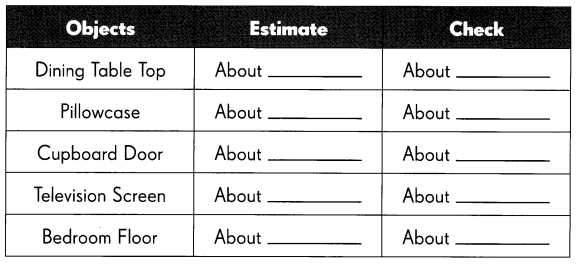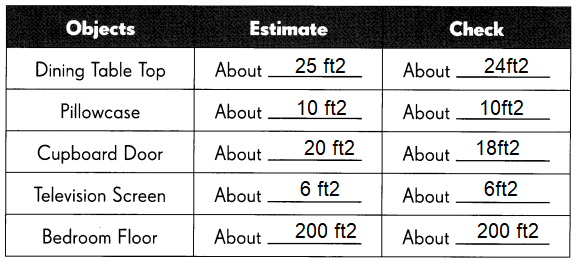# Math in Focus Grade 3 Chapter 19 Practice 3 Answer Key Square Units (m² and ft²)

This handy Math in Focus Grade 3 Workbook Answer Key Chapter 19 Practice 3 Square Units (m² and ft²) detailed solutions for the textbook questions.

## Math in Focus Grade 3 Chapter 19 Practice 3 Answer Key Square Units (m² and ft²)

Find the area of each shaded figure in square meters. Then complete the table.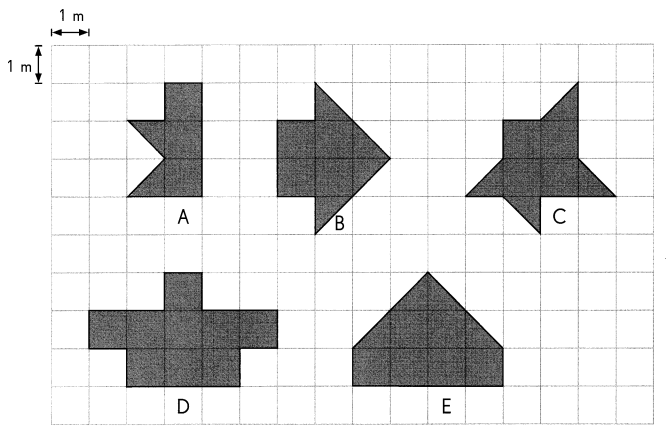Question 1.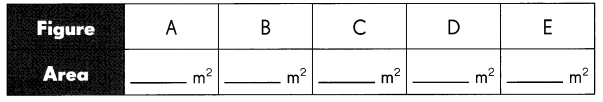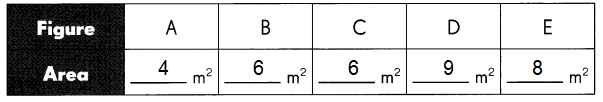Explanation:
The simplest (and most commonly used) area calculations are for squares and rectangles.
To find the area of a rectangle, multiply its height by its width.
For a square you only need to find the length of one of the sides (as each side is the same length) and,
then multiply this by itself to find the area.

Question 2.
Which figure has the smallest area? Figure ____________
Figure A
Explanation:
Compare all the figures given in the grid,
figure A has smallest area 4 square meters.

Question 3.
Which figure has the largest area? Figure ____________
Figure D
Explanation:
Compare all the figures given in the grid,
figure D has greatest area 9 square meters.

Question 4.
Which figures have the same area? Figures ____________
Figure B & Figure C
Explanation:
Compare all the figures given in the grid,
figure A and B has the same area 6 square meters.

Find the area of each shaded figure in square feet. Then complete the table.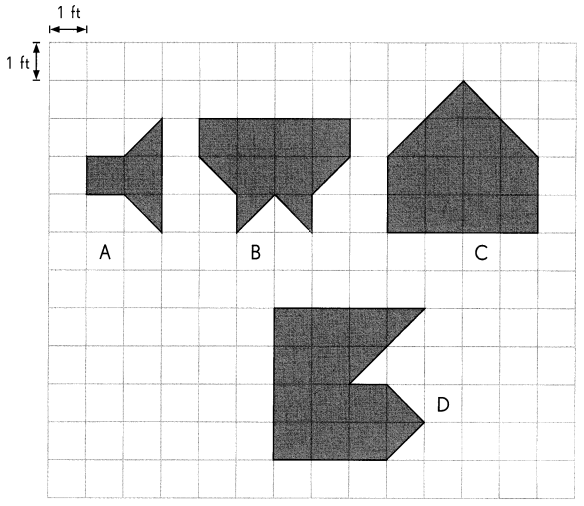Question 5.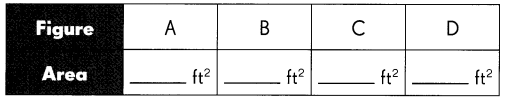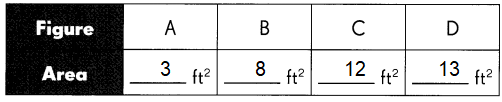Explanation:
The simplest (and most commonly used) area calculations are for squares and rectangles.
To find the area of a rectangle, multiply its height by its width.
For a square you only need to find the length of one of the sides (as each side is the same length) and,
then multiply this by itself to find the area.

Question 6.
Which figure has the largest area? Figure ____________
Figure D
Explanation:
Compare all the figures given in the grid,
figure D has largest area 13 square feet.

Question 7.
Which figure has the smallest area? Figure _____________
Figure A
Explanation:
Compare all the figures given in the grid,
figure A has smallest area 3 square feet.

The figures are made of square and half-square tiles. Find the area of each shaded figure. Then complete the table.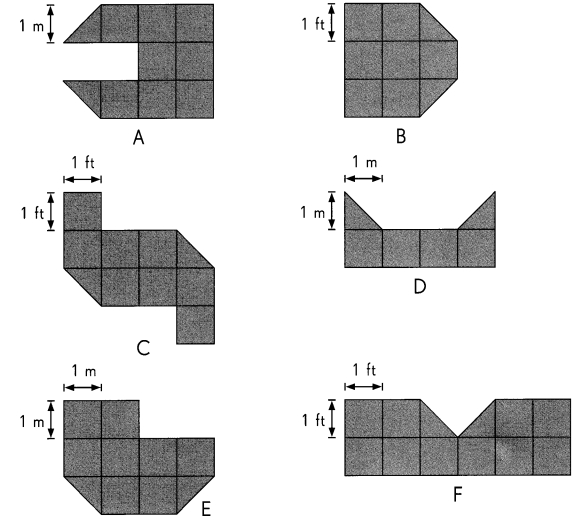Question 8.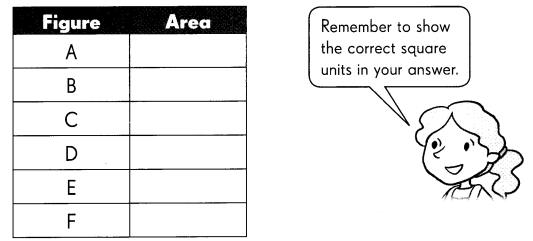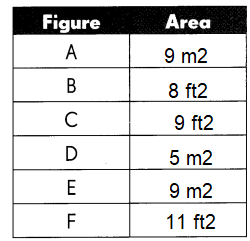Explanation:
The simplest (and most commonly used) area calculations are for squares and rectangles.
To find the area of a rectangle, multiply its height by its width.
For a square you only need to find the length of one of the sides (as each side is the same length) and,
then multiply this by itself to find the area.

Make square pieces of paper with an area of 1 square meter and 1 square foot. Use a ruler and scissors to cut out the squares.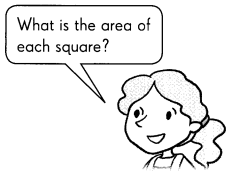Estimate the area in square feet.

Question 9.
Explanation:
Measurements may vary from one to one,
depending upon the size of the kitchen.
So, we use the square meter to estimate the area of the kitchen.
Question 10.
Explanation:
Measurements may vary from one to one,
depending upon the size of the bed.
So, we use the square feet to estimate the area of the bed.

Use the square meter and square foot paper to estimate the area of these objects in your house.

Question 11.